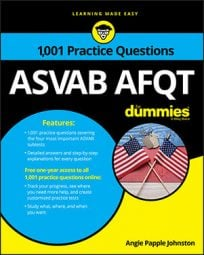##### ASVAB AFQTIf you love circles—and who doesn't!—then you're in for a treat when you take the Mathematics Knowledge subtest on the ASVAB. Of course, enthusiasm isn't enough; you should also know the formulas for the circumference and area of a circle, and also how to switch a formula around to get the right answer.

## Practice questions

1. Based on this diagram,

2. Based on this diagram,

find the circumference of the circle.

1. The correct answer is Choice (B).

The formula for the area of a circle is

2. The correct answer is Choice (C).

First, notice that the answer choices are in terms of pi. That means you won't have to multiply by 3.14.

The formula to find the circumference of a circle is

Because you know the diameter is 210 feet, the radius is 105 feet. Use what you know in the formula:

You can solve the problem more quickly using an alternate formula for the circumference,

where d is the diameter. Mentally insert d = 210 in for d, and you get